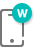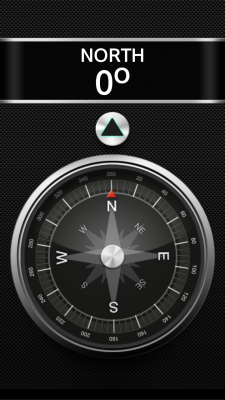# Compass Sample OverviewThe Compass sample application demonstrates how you can use the W3C DeviceOrientation Event in your application to monitor the device orientation.

The following figure illustrates the main screen of the Compass.

Figure: Compass screenThe application opens with the main screen that shows the current device orientation.

## Source Files

You can create and view the sample application project including the source files in the IDE.

File name Description
config.xml This file contains the application information for the platform to install and launch the application, including the view mode and the icon to be used in the device menu.
index.html This is a starting file from which the application starts loading. It contains the layout of the application screens.
js/main.js This file contains the code for handling the device orientation functionality of the application.

## Implementation

The init() method, which is called when the whole DOM content is loaded, initializes the application. It defines three references to the UI elements and calls the bindEvents() methods.

```/* main.js */
var directionEl = null,
angleEl = null,
rotationEl = null;

function init()
{
directionEl = document.getElementById('direction');
angleEl = document.getElementById('angle');
rotationEl = document.getElementById('rotation');
bindEvents();
}

```

The bindEvents() method defines handler methods for tizenhwkey and deviceorientation events. The first one uses the exit() method and allows user to close application using device hardware back button. The other one defines the onDeviceOrientation() method as a callback.

```/* main.js */
function bindEvents()
{
{
if (e.keyName === 'back')
{
exit();
}
});
}
```

The onDeviceOrientation() method is responsible for updating the application UI (displayed degree and direction values and compass rotation animation) according to the information obtained from the W3C DeviceOrientation event. It takes an event object describing the physical orientation of the device. To get the compass heading data, the application uses the alpha parameter of this object. The alpha parameter provides a value expressed in degrees (ranged from 0 to 360) and allows easily calculating and displaying the correct direction.

Another task of the onDeviceOrientation() method is to calculate the rotation of the compass. The application uses the lastAngle variable to store previously measured alpha angle in order to calculate the value of deltaAngle variable which represents the value change. Depending on the deltaAngle value, the application calculates the value of the rotation variable to provide the shortest path for the compass UI animation. The compass UI is animated using the -webkit-transform CSS property.

```/* main.js */
var lastAngle = 0, /* Last measurement */
rotation = 0; /* Compass rotation */

function onDeviceOrientation(eventData)
{
var angle = eventData.alpha,
deltaAngle,
text = '';

/* Check whether the angle has changed since the last measurement */
if (isNaN(eventData.alpha) ||
eventData.alpha === lastAngle)
{
return;
}

/* Find direction from a given angle */
if (angle < 68 || angle > 292)
{
text = 'NORTH';
}
else if (angle > 112 && angle < 248)
{
text = 'SOUTH';
}
if (angle > 22 && angle < 158)
{
text += 'EAST';
}
else if (angle > 202 && angle < 338)
{
text += 'WEST';
}

/* Calculate the rotation of the compass */
deltaAngle = lastAngle - angle;
if (deltaAngle > 180)
{
rotation -= 360 - deltaAngle;
}
else if (deltaAngle < -180)
{
rotation += 360 + deltaAngle;
}
else
{
rotation += deltaAngle;
}

/* Save the current value */
lastAngle = angle;

/* Update the direction and angle labels */
directionEl.innerHTML = text;
angleEl.innerHTML =
Math.round(angle) + '°';
/* Rotate the compass image */
rotationEl.style['-webkit-transform'] = 'rotate(' + rotation + 'deg)';
}
```